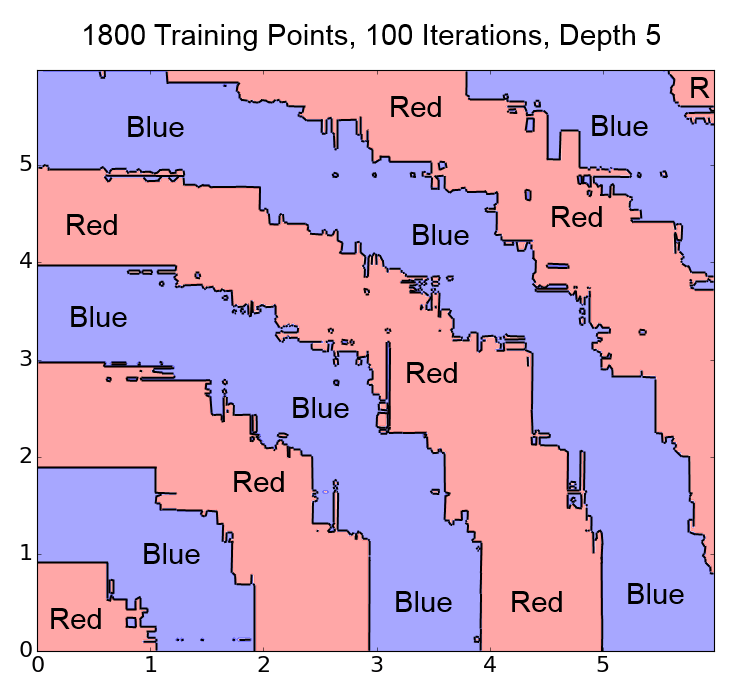## Boosting Examples

### Gradient Boosted Trees Programs & Excel Examples Of Key Equations

This zip file contains several different Python Programs utilizing easy to understand examples for regression and classification gradient boosted trees.  Those examples include training and testing the data as well as some examples of feature generation.

Also included is an Excel file that has a lot of useful information on how Gradient Boosted Trees work, such as the equations that they use to calculate the change in each data point for each boosting iteration.

50% Complete

You're Almost there! Please complete this form and click the button below to gain instant access.• A Python program that uses gradient boosted trees for regression, and also does the same regression math without Sklearn
• Another python program that does boosting for classification using the different colored stripes shown in the book
• An Excel file that contains the examples for how to do boosting calculations and other information